# Math in Focus Grade 8 Chapter 5 Review Test Answer Key

Practice the problems of Math in Focus Grade 8 Workbook Answer Key Chapter 5 Review Test to score better marks in the exam.

## Math in Focus Grade 8 Course 3 A Chapter 5 Review Test Answer Key

Concepts and Skills

Solve each system of linear equations using the elimination method.

Question 1.
3x + 2y = 18
2x + 3y = 22
3x + 2y = 18 Equation 1
2x + 3y = 22 Equation 2
We are given the system of equations:
2 ∙ (3x + 2y) = 2 ∙ 18 We can eliminate first either x, or y.
6x + 4y = 36 Equation 3 We usually choose to eliminate the variable which has smaller coefficients, in this case both have the same coefficients. We choose to eliminate x.
Multiply Equation 1 by 2:
3 ∙ (2x + 3y) = 3 ∙ 22 Multiply Equation 2 by 3:
6x + 9y = 66 Equation 4
(6a + 9y — (6x + 4y) = 66 — 36 We subtract Equation 3 from Equation 4:
6x + 9y — 6x — 4y = 30 Use the distributive property:
5y = 30 Simplify, 6x is eliminated:
$$\frac{5 y}{5}$$ = $$\frac{30}{5}$$ Divide by 5 and simplify:
y = 6
3x + 2(6) = 18 Substitute 6 for y in Equation 1:
3x + 12 = 18 Simplify:
Subtract 12 from both sides:
3x + 12 — 12 = 18 — 12
3x = 6 Simplify:
$$\frac{3 x}{3}$$ = $$\frac{6}{3}$$ Divide by 3:
x = 2
y = 6 The solution to the system of linear equations is
x = 2
y = 6

Question 2.
5x + y = 8
x + 3y = 10
5x + y = 8 Equation 1 We are given the system of equations:
x + 3y = 10 Equation 2
3 ∙ (5x + y) = 3 ∙ 8 We can eliminate first either x, or y.
15x + 3y = 24 Equation 3 We usually choose to eliminate the variable which has smaller coefficients, in this case y. Multiply Equation 1 by 3:
(15x + 3y) – (x + 3y) = 24 – 10 We subtract Equation 2 from Equation 3:
15x + 3y – x – 3y = 14 Use the distributive property:
14x = 14 Simplify, 3y is eliminated:
$$\frac{14 x}{14}$$ = $$\frac{14}{14}$$
Divide by 14 and simplify:
x = 1
5(1) + y = 8 Substitute 1 for x in Equation 1:
5 + y = 8 Simplify:
5 + y – 5 = 8 – 5 Subtract 5 from both sides:
y = 3 Simplify:
x = 1
The solution to the system of linear equations is
y = 3
x = 1; y = 3

Question 3.
$$\frac{1}{2}$$a + b = 7
a + 3b = 19
$$\frac{1}{2}$$a + b = 7 Equation 1
a + 3b = 19 Equation 2
We are given the system of equations:
2 ∙ ($$\frac{1}{2}$$a + b) = 7 We can eliminate rirst either a, or b
a + 2b = 14 Equation 3 We usually choose to etiminate the variable which has smaller coefficients, in this case a.
Multiply Equation 1 by 2:
(a + 3b) — (a + 2b) = 19 — 14 We subtract Equation 3 from Equation 2:
a + 3b — a — 2b = 5 Use the distributive property
b = 5 Simplify, a is eliminated:
a + 3(5) = 19 Substitute 5 for b in Equation 2:
a + 15 = 19 Simplify:
a + 15 — 15 = 19 — 15 Subtract 15 from both sides:
a = 4 Simplify:
a = 4 The solution to the system of linear equations is
b = 5
a = 4 ; b = 5

Solve each system of linear equations using the substitution method.

Question 4.
a + 2b = 1
2a + b = 8
a + 2b = 1 Equation 1 We are given the system of equations:
2a + b = 8 Equation 2
2a + b = 8 Use Equation 2 to express b in terms of a:
2a + b — 2a = 8 — 2a Subtract 2a from both sides:
b = 8 — 2a Equation 3 Simplify:
a + 2(8 — 2a) = 1 Substitute Equation 3 into Equation 1:
a + 16 — 4a = 1 Use the distributive property:
—3a + 16 = 1 Simplify:
—3a + 16 — 16 = 1 — 16 Subtract 16 from both sides:
—3a = —15 Simplify:
$$\frac{-3 a}{-3}$$ = $$\frac{-15}{-3}$$ Divide by -3:
a = 5 Simplify:
b = 8 — 2(5) Substitute 5 for a in Equation 3:
= 8 — 10
= -2
a = 5 The solution of the system ¡S:
b = -2
a = 5; b = -2

Question 5.
2x + 11y = 15
x – y = 1
2x + 11y = 15 Equation 1 We are given the system of equations:
x — y = 1 Equation 2
x — y = 1 Use Equation 2 to express x in terms of y:
x – y + y = 1 + y Add y to both sides:
x = y + 1 Equation 3 Simplify:
2(y + 1) + 11y = 15 Substitute Equation 3 into Equation 1:
2y + 2 + 11y = 15 Use the distributive property:
13y + 2 = 15 Simplify:
13y + 2 — 2 = 15 — 2 Subtract 2 from both sides:
13y = 13 Simplify:
$$\frac{13 y}{13}$$ = $$\frac{13}{13}$$ Divide by 13:
y = 1 Simplify:
x = 1 + 1
= 2 Substitute 1 for y in Equation 3:
x = 2 The solution of the system is:
y = 1
x = 2
y = 1

Question 6.
$$\frac{1}{2}$$x + $$\frac{1}{2}$$y = 7
3x – y = 22
We are given the system of equations:
$$\frac{1}{2}$$x + $$\frac{1}{2}$$y = 7 Equation 1
3x — y = 22 Equation 2
3x — y = 22 use Equation 2 to express y is terms of x:
3x — y — 3x = 22 — 3x Subtract 3x from both sides:
—y = 22 — 3x Simplify:
y = 3x — 22 Equation 3
$$\frac{1}{2}$$x + $$\frac{1}{2}$$(3x – 22) Substitute Equation 3 into Equation 1:
2 • $$\frac{1}{2}$$x + 2 • $$\frac{1}{2}$$(3x —22) = 2 • 7 Multiply both sides by 2:
x + 3x — 22 = 14 Simplify:
4x — 22 = 14
4x — 22 + 22 = 14 + 22 Add 22 to both sides:
4x = 36 Simplify:
$$\frac{4 x}{4}$$ = $$\frac{36}{4}$$ Divide by 4
x = 9 Simplify:
y = 3(9) — 22 Substitute 9 for x in Equation 3:
= 27 — 22
= 5
x = 9 The solution of the system is:
y = 5
x = 9
y = 5

Solve each system of linear equations using the graphical method. Use 1 grid square to represent 1 unit on both axes for the interval -3 to 8.

Question 7.
2x + 3y = 24
2x + y = 12
2x + 3y = 24 Equation 1
2x + y = 12 Equation 2
We are given the system of equations:
2x + 3y = 24 We rewrite Equation 1 in slope-intercept form:
2x + 3y – 2x = 24 — 2x
3y = —2x + 24
$$\frac{3 y}{3}$$ = $$\frac{-2 x+24}{3}$$
y = –$$\frac{2}{3}$$x + 8
2x + y = 12 we rewrite Equation 2 in slope-intercept rorm:
2x + y – 2x = 12 — 2x
y = —2x + 12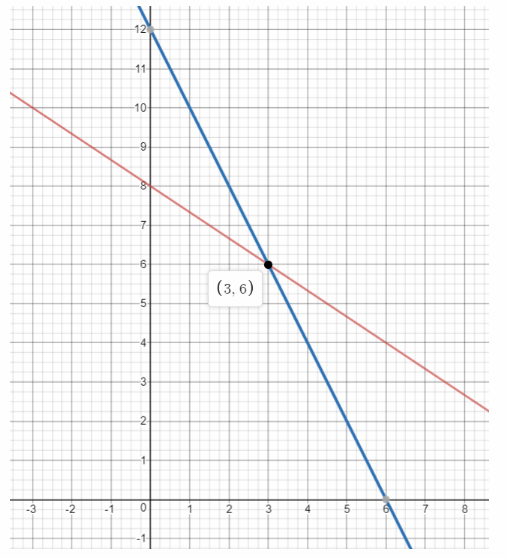We graph the two equations: the first has slope —$$\frac{2}{3}$$ and y-intercept 8, while the second has slope -2 and y-intercept 12:
x = 3 The point of intersection is (3, 6). Therefore the solution of the system of equations is
y = 6
x = 3; y = 6

Question 8.
2x + 5y = 1
3x – y = – 7
2x + 5y = 1 Equation 1
3x – y = —7 Equatiou 2
We are given the system of equations:
2x + 5y = 1 We rewrite Equation 1 in slope-intercept form:
2x + 5y — 2x = 1 — 2x
5y= —2x + 1
$$\frac{5 y}{5}$$ = $$\frac{-2 x+1}{5}$$
y = –$$\frac{2}{5}$$x + $$\frac{1}{5}$$
3x — y = —7 We rewrite Equation 2 in slope-intercept form:
3x — y — 3x = —7 — 3x
—y = —3x — 7
y = 3x + 7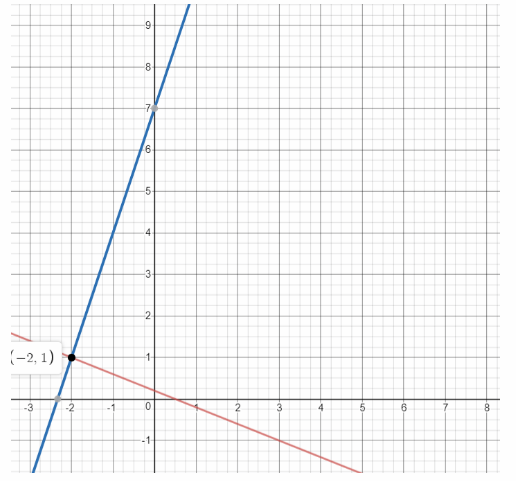We graph the two equations: the first has slope —$$\frac{2}{5}$$ and y-intercept $$\frac{1}{5}$$, while the second has slope 3 and y-intercept 7:
x = -2 The point of intersection is (—2, 1). Therefore the solution of the system of equations is:
y = 1
x = —2; y = 1

Question 9.
x + 0.5y = 6
3x – y = 13
x + 0.5y = 6 Equation 1
3x — y = 13 Equation 2
We are given the system of equations:
x + 0.5y = 6 We rewrite Equation 1 in stope-intercept form:
x + 0.5y — x = 6 — x
0.5y = —x + 6
$$\frac{0.5 y}{0.5}$$ = $$\frac{-x+6}{0.5}$$
y = -2x + 12
3x — y = 13 We rewrite Equation 2 in slope-intercept form:
3x — y — 3x = 13 — 3x
—y = —3x + 13
y = 3x — 13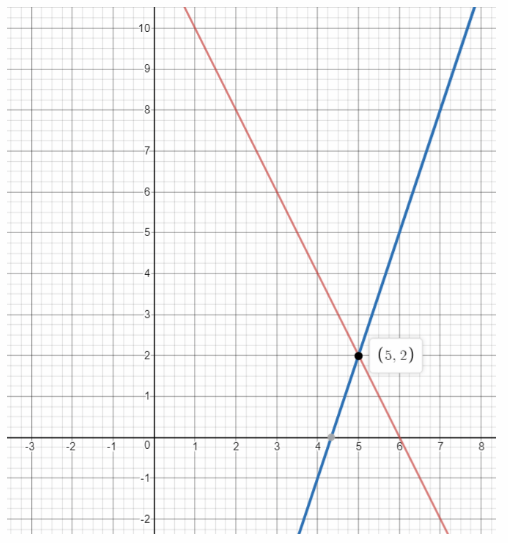We graph the two equations: the first has slope -2 and y-intercept 12. while the second has slope 3 and y-intercept -13:
x = 5 The point of intersection is (5, 2). Therefore the solution of the system of equations is
y = 2
x = 5; y = 2

Solve each system of linear equations. Explain your choice of method.

Question 10.
x = 4y – 1
2x – 6y = – 1
x = 4y — 1 Equation 1 We are given the system of equations:
2x — 6y = —1 Equation 2
5x + y = 15 As there is a variable with coefficient 1 (which is x in Equation 1), we can use the substitution method.
x = 4y — 1 Equation 1 expresses x is terms of y:
2(4y – 1) – 6y = —1 Substitute Equation 1 into Equation 2:
8y — 2 – 6y = —1 Use the distributive property
2y — 2 = —1 Simplify:
2y — 2 + 2 = —1 + 2 Add 2 to both sides:
2y = 1 Simplify:
$$\frac{2 y}{2}$$ = $$\frac{1}{2}$$ Divide by 2:
y = $$\frac{1}{2}$$ simplify
x = 4($$\frac{1}{2}$$) – 1 Substitute $$\frac{1}{2}$$ for y in Equation 1:
= 2 – 1
= 1
x = 1 The solution of the system is
y = $$\frac{1}{2}$$
x = 1
y = $$\frac{1}{2}$$

Question 11.
3x – 14y = -49
5x + 2y = 45
3x — 14y = -49 Equation 1
5x + 2y = 45 Equation 2
We are given the system of equations:
7 • (5x + 2y) = 7 . 45 The substitution method would lead to fractions as none of the variables has coefficient 1.
35x + 14y = 315 Equation 3
We use the elimination method.
We can eliminate first either z, or g.
We choose to eliminate the variable y because -14 is multiple of 2.
Multiply Equation 2 by 7:
(3x — 14y) + (35x + 14y) = —49 + 315 We add Equation 3 to Equation 1:
3x — 14y + 35x + 14y = 266 Simplify, 14y is eliminated:
38x = 266
$$\frac{38 x}{38}$$ = $$\frac{266}{38}$$ Divide by 38 and simplify
x = 7
5(7) + 2y = 45 Substitute 7 for x in Equation 2:
35 + 2y = 45 Simplify:
35 + 2y — 35 = 45 — 35 Subtract 35 from both sides:
2y = 10 Simplify:
$$\frac{2 y}{2}$$ = $$\frac{10}{2}$$ Divide by 2
y = 5 simplify
x = 7 The solution to the system of linear equations is
y = 5
x = 7
y = 5

Question 12.
x – $$\frac{1}{3}$$y = 6
2x + y = 2
x – $$\frac{1}{3}$$y = 6 Equation 1
2x + y = 2 Equation 2
We are given the system of equations:
x – $$\frac{1}{3}$$y = 6 We can use substitution or graphical method as we have at least one variable with coefficient 1. We choose the graphical method
x – $$\frac{1}{3}$$y – x = 6 – x
–$$\frac{1}{3}$$y = -x + 6 We rewrite Equation 1 in slope-intercept form:
—3($$\frac{1}{3}$$y) = —3(—x + 6)
y = 3x— 18
2x + y = 2 We rewrite Equation 2 in slope-intercept form:
2x + y — 2x = 2— 2x
y = —2x + 2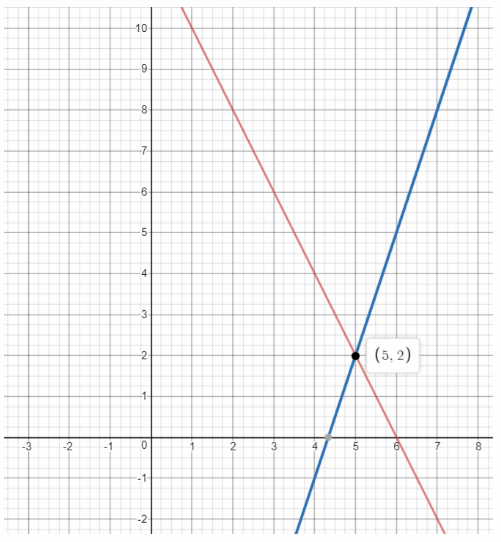We graph the two equations: the first has slope 3 and y-intercept -18, while the second has slope -2 and y-intercept 2:
x = 5 The point of intersection is (5, 2). Therefore the solution of the system of equations is
y = 2
x = 5
y = 2

Identify whether each system of equations is inconsistent, dependent, or has a unique solution. Justify your answer. Solve the system of linear equations if it has a unique solution.

Question 13.
3x + 2y = 8
6x + 4y = 16
3x + 2y = 8 Equation 1
6x + 4y = 16 Equation 2
we are given the system of equations
3x + 2y = 8 Rewrite Equation 1 in slope-intercept form y = mx + b
3x + 2y – 3x = 8 – 3x Subtract 3x from both sides and simplify
2y = -3x + 8
$$\frac{2 y}{2}$$ = $$\frac{-3 x+8}{2}$$ Divide both sides by 2 and simplify
y = $$-\frac{3}{2} x$$ + 4
6x + 4y = 16 Rewrite Equation 2 in slope-intercept form y = mx + b:
6x + 4y — 6x = 16 — 6x Subtract 6x from both sides and simplify:
4y = —6x + 16
$$\frac{4 y}{4}$$ = $$\frac{-6 x+16}{4}$$ Divide both sides by 4 and simplify:
y = $$-\frac{3}{2} x$$ + 4
Since Equation 1 and Equation 2 are equivalent, they have an infinite number of solutions.
Therefore system of linear equations is dependent
Dependent

Question 14.
$$\frac{x}{3}$$ + y = 8
x + 3y = 10
$$\frac{1}{3} x$$ + y = 8 Equation 1
x + 3y = 10 Equation 2
We are given the system of equations:
$$\frac{1}{3} x$$ + y = 8
Rewrite Equation 1 in slope-intercept form y = mx + b:
$$\frac{1}{3} x$$ + y – $$\frac{1}{3} x$$ = 8 – $$\frac{1}{3} x$$ Subtract $$\frac{1}{3} x$$ from both sides and simplify:
y = –$$\frac{1}{3} x$$ + 8
x + 3y = 10 Rewrite Equation 2 in slope-intercept form y = mx + b
x + 3y — x = 10 — x Subtract x from both sides and simplify:
$$\frac{3 y}{3}$$ = $$\frac{-x+10}{3}$$ Divide both sides by 3 and simplify:
y = $$-\frac{1}{3} x$$ + $$\frac{10}{3}$$
The linear equations have the same slope and different y-intercept. The system of linear equations is inconsistent because the system of Linear equations has no solution.
Inconsistent

Question 15.
$$\frac{1}{2}$$x + y = 7
x + 2y = 14
$$\frac{1}{2}$$x + y = 7 Equation 1
x + 2y = 14 Equation 2
We are given the system of equations:
$$\frac{1}{2}$$x + y = 7 Rewrite Equation 1 in slope-intercept form y = mx + b:
$$\frac{1}{2}$$x + y — $$\frac{1}{2}$$x = 7 — $$\frac{1}{2}$$x Subtract $$\frac{1}{2}$$x from both sides and simplify:
y = –$$\frac{1}{2}$$x + 7
x + 2y = 14 Rewrite Equation 2 in slope-intercept form y = mx + b:
x + 2y — x = 14 — x Subtract x from both sides and simplify:
$$\frac{2 y}{2}$$ = $$\frac{-x+14}{2}$$ Divide both sides by 2 and simplify:
Since Equation 1 and Equation 2 are equivalent they have an infinite number of solutions.
Therefore system of linear equations is dependent.
Dependent

Question 16.
2x – $$\frac{1}{2}$$y = 10
y = 4x + 11
2x — $$\frac{1}{2}$$y = 1o Equation 1
y = 4x + 11 Equation 2
We are given the system of equations:
2x – $$\frac{1}{2}$$y = 10 Rewrite Equation 1 in slope-intercept form y = mx + b:
2x – $$\frac{1}{2}$$y – 2x = 10 – 2x Subtract 2x from both sides and simplify:
–$$\frac{1}{2}$$y = -2x + 10
-2 ∙ (-$$\frac{1}{2}$$y) = -2(-2x + 10) Multiply both sides by -2 and simplify
y = 4x — 20
y = 4x + 11 Equation 2 is written in slope-intercept form y = mx + b
The linear equations have the same slope and different y-intercept The system of linear equations is inconsistent because the system of linear equations has no solution.
Inconsistent

Question 17.
2x – 5y = -21
4x + 3y = 23
2x — 5y = —21 Equation 1
4x + 3y = 23 Equation 2
We are given the system of equations:
2(2x — 5y) = 2(—21) We use the elimination method.
4x — 10y = —42 Equation 3 Multiply Equation 1 by 2:
(4x + 3y) — (4x — 10y) = 23 — (—42) We subtract Equation 3 from Equation 2 and simplify:
4x + 3y — 4x + 10y = 65
13y = 65
$$\frac{13 y}{13}$$ = $$\frac{65}{13}$$ Divide by 13 and simplify:
y = 5
4x + 3(5) = 23 Substitute 5 for y in Equation 2:
4x + 15 = 23
4x + 15 — 15 = 23 — 15 Subtract 15 from both sides and simplify:
4x = 8
The system of linear equations has an unique solution:
$$\frac{4 x}{4}$$ = $$\frac{8}{4}$$ Divide by 4 and simplify:
x = 2
x = 2 The system of Linear equations has an unique solution:
y = 5
Unique solution (x = 2, y = 5)

Question 18.
6y – 12x = 60
4y – 40 = 8x
6y — 12x = 60 Equation 1
4y — 40 = 8x Equation 2
We are given the system of equations:
6y — 12x = 60 Rewrite Equation 1 in slope-intercept form y = mx + b:
6y — 12x + 12x = 60 + 12x Add 12x to both sides and simplify
6y = 12x + 60
$$\frac{6 y}{6}$$ = $$\frac{12 x+60}{6}$$ Divide both sides by 6 and simplify:
y = 2x + 10
4y – 40 = 8x Rewrite Equation 2 in slope-intercept form y = mx + b
4y — 40 + 40 = 8x + 40 Add 40 to both sides and simplify:
4y = 8x + 40
$$\frac{4 y}{4}$$ = $$\frac{8 x+40}{4}$$ Divide both sides by 4 and simplify:
y = 2x + 10
Since Equation 1 and Equation 2 are equivalent, they have an infinite number of solutions. Therefore system of linear equations is dependent
Dependent

Problem Solving

Question 19.
Andy and Ben both worked a total of 88 hours one week. Ben worked 8 hours more than Andy. Find the number of hours each man had worked.
Lets note:
a = the number of hours worked by Andy
b = the number of hours worked by Ben
a + b = 88 Equation 1 We write the system of equations:
b = a + 8 Equation 2
We use the substitution method.
b is expressed in terms of a in Equation 2:
a + (a + 8) = 88 Substitute Equation 2 into Equation 1:
a + a + 8 = 88 Simplify:
2a +8 = 88
2a + 8 – 8 = 88 — 8 Subtract 8 from both sides:
2a = 80 Simplify:
$$\frac{2 a}{2}$$ = $$\frac{80}{2}$$ Divide both sides by 2:
a = 40 simplify
b = 40 + 8 Substitute 40 for a in Equation 2:
= 48
So Andy worked 40 hours and Ben worked 48 hours
Andy: 40 hours
Ben: 48 hours

Question 20.
In three years’ time, Mr. Sullivan will be 3 times as old as his daughter. Six years ago, he was 6 times as old as she was. How old are they now?
s + 3 = 3(d + 3) Equation 1
s — 6 = 6(d — 6) Equation 2
Lets note:
s = Mr Sullivans age now
d = the daughters age now
We can write the system of equations:
s — 6 = 6d — 36 We use the substitution method.
s — 6 + 6 = 6d — 36 + 6 Rewrite Equation 2 to express s in terms of d:
s = 6d — 30 Equation 3
(6d — 30) + 3 = 3(d + 3) Substitute Equation 3 in Equation 1 and simplify:
6d — 30 + 3 = 3d + 9
6d — 27 = 3d + 9
6d — 27 – 3d = 3d + 9 – 3d We subtract 3d from both sides:
3d — 27 = 9
3d — 27 + 27 = 9 + 27 Add 27 to both sides:
3d = 36
$$\frac{3 d}{3}$$ = $$\frac{36}{3}$$ Divide by 3 and simplify:
d = 12
s = 6(12) — 30 Substitute 12 for d in Equation 3:
= 72 – 30
= 42
d = 12 The solution to the system of linear equations is:
s = 42
Mr Sullivan’s age is 42 years, while his daughter’s is 12 years now
Mr Sullivan: 42 years
The daughter: 12 years

Question 21.
Samantha has a riddle for her sister: Find a pair of integers x and y that satisfy 7x + 3y = 64 and one of the integers is 3 times the other.
7x + 3y = 64 Equation 1
x = 3y Equation 2
We write the systems of equations:
7x + 3y = 64 Equation 1
y = 3x Equation 2
x = 3y Equation 1 We solve the first system using substitution.
7(3y) + 3y = 64 Substitute Equation 2 into Equation 1:
21g + 3y = 64
24y = 64
$$\frac{24 y}{24}$$ = $$\frac{64}{24}$$ We determine y:
y = $$\frac{8}{3}$$
As y is not integer, this is not a solution.
y = 3x Equation 2 We solve the second system using substitution.
7x + 3(3x) = 64 Substitute Equation 2 into Equation 1:
7x + 9x = 64
16x = 64
$$\frac{16 x}{16}$$ = $$\frac{64}{24}$$ We determine x:
x = 4
y = 3(4) Substitute 4 for x in Equation 2:
= 12
x = 4 The two integers are 4 and 12.
y = 12
x = 4
y = 12

Question 22.
The shape ABC in the quilt block below is an isosceles triangle. In triangle ABC, AB = AC. The perimeter of triangle ABC is 27.3 inches. Find the values of x and y. Then find the length of each side of the triangle in inches.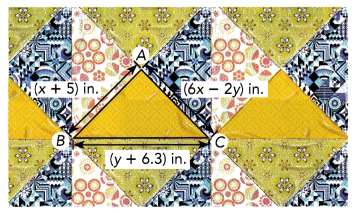x + 5 = 6x — 2y Equation 1
2(x + 5) + y + 6.3 = 27.3 Equation 2 We can write the system of equations:

x + 5 – x = 6x — 2y — x Equation 1
2x + 10 + y + 6.3 = 27.3 Equation 2
we bring me system to the standard form:

5x — 2y = 5 Equation 1
2x + y + 16.3 = 27.3 Equation 2

5x — 2y = 5 Equation 1
2x + y + 16.3 — 16.3 = 27.3 — 16.3 Equation 2

5x — 2y = 5 Equation 1
2x + y = 11 Equation 2

2x + y = 11 We use the substitution method.
2x + y — 2x = 11 — 2x We express y in terms of x from Equation 2:
y = —2x + 11 Equation 3
5x — 2(—2x + 11) = 5 Substitute Equation 3 into Equation 1:
5x + 4x — 22 = 5
9x — 22 = 5
9x — 22 + 22 = 5 + 22 We determines x:
9x = 27
$$\frac{9 x}{9}$$ = $$\frac{27}{9}$$
x = 3
y = —2(3) + 11 Substitute 3 for x in Equation 3:
= -6 + 11
= 5
x = 3 The system’s solution is:
y = 4
AB = AC = x + 5 = 3 + 5 = 8 We determine AB and AC:
BC = y + 6.3 = 5 + 6.3 = 11.3 We determine BC:
x = 3; y = 5
AB = 8 inches; AC = 8 inches BC = 11.3 inches

Question 23.
A bus company requires 4 buses and 8 vans to take 240 school children to the library. It requires 2 buses and 9 vans to take 170 children to the museum. Calculate the number of children a bus can carry and the number of children a van can carry.
Let’s note:
b = the number of children in a bus
v = the number of children in a van
4b + 8v = 240 Equation 1
26 + 9v = 170 Equation 2
We write the system of equations:
2(2b + 9v) = 2(170) We use the elimination method.
4b + 18v = 340 Equation 3 Multiply Equation 2 by 2:
(4b + 18v) — (4b + 8v) = 340 — 240 Subtract Equation 1 from Equation 3:
4b + 18v — 4b — 8v = 100 Simplify:
10v = 100
$$\frac{10 v}{10}$$ = $$\frac{100}{10}$$ Divide by 10 and simplify:
v = 10
2b + 9(10) = 170 Substitute 10 for v in Equation 2:
2b + 90 = 170
2b + 90 — 90 = 170 — 90 Subtract 90 from both sides:
2b = 80
$$\frac{2 b}{2}$$ = $$\frac{80}{2}$$ Divide both sides by 2:
b = 40 Simplify:
A bus can carry 40 children, while a van can carry 10 children.
Bus: 40 children
Van : 10 children

Question 24.
At noon, Balloon M is 60 meters above ground, and Balloon N is 50 meters above ground. Balloon M is rising at the rate of 10 meters per second, while Balloon N is rising at the rate of 15 meters per second.

a) Write a system of two linear equations in which each equation gives the height, h meters, of a balloon t seconds after noon. Then solve the system using a graphing calculator.
h = 60 + 10t Equation 1 a) We write a system of equations giving the height of each balloon:
h = 50 + 15t Equation 2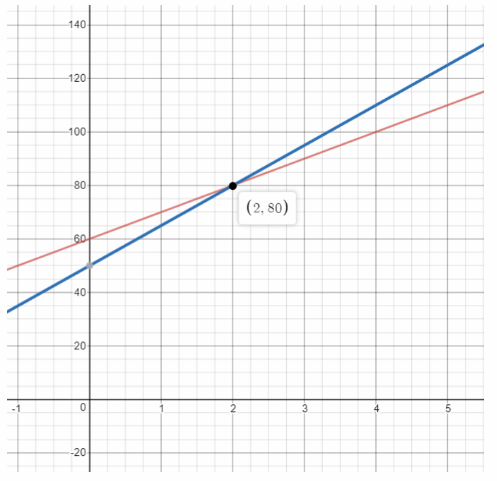We use a graphing calculator to solve the system:
t = 2 The solution is:
h = 80

b) How many seconds after noon will the two balloons be at the same height?
How do you know?
t = 2 seconds b) The two balloons will be at the same height when h = 80. We determined the time when this happens, which is the t-coordinate of the solution above:
a) t = 2, h = 80
b) 2 seconds

Question 25.
The water levels in two identical tanks rise at a rate of 4 inches per second. The water level, h inches, in Tank A is 3 inches at t = 0. The water level in Tank B is 16 inches at t = 3 seconds.

a) Write a system of equations for the water level h in the two tanks in terms of t.
h = 3 + 4t Equation 1 a) we write system of equations for the water level in the two tanks
h = 16 + 4(t – 3) Equation 2

b) Graph the two equations on the same coordinate plane. Use 1 grid square on both axes to represent 1 unit for the interval 0 to 10.
h = 4t + 3 Equation 1 b) We write the equations in slope-Intercept form
h = 16 + 4t – 12 Equation 2
h = 4t + 3 Equation 1
h = 4t + 4 Equation 2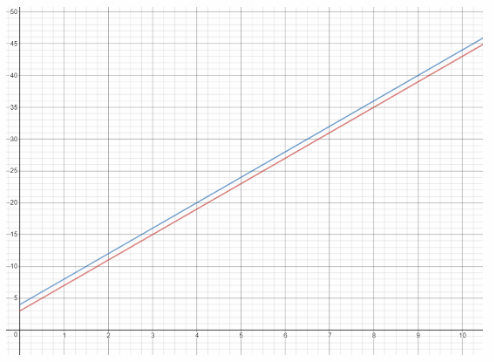We graph the two equations the first has slope 4 and y-Intercept 3, while the second has slope 4 and y-intercept 4.

c) When will the two tanks have the same water level? How do you know?
The two tanks will never have the same level because the system of equations in part (a) is inconsistent because the slope is the same, but the y-intercept is not

a)
h = 3 + 4t
h = 16 + 4(t — 3)
b) See graph
c) Never

Question 26.
Natalie has x bags of onions and y bags of potatoes. There are a total of 8 bags. Each bag weighs 2 pounds. The total weight of the bags is 16 pounds. She wants to find the value of x and y.

a) Write a system of two linear equations.
x + y = 8 Equation 1
2x + 2y = 16 Equation 2
a) We write a system of equations:

b) State with reasons whether the system of equations has a unique solution, is inconsistent, or is dependent.
$$\frac{1}{2}$$ = $$\frac{1}{2}$$ = $$\frac{8}{16}$$ b) We notice that the coefficients of the variables and the constants are proportional: